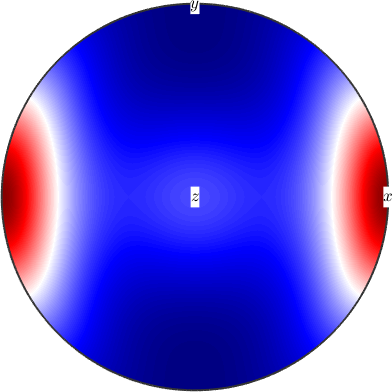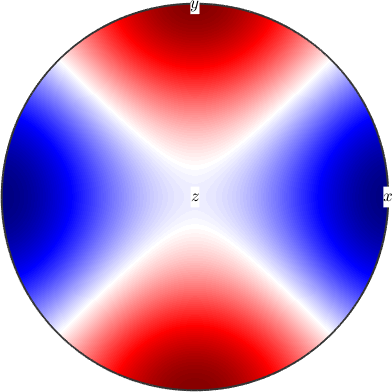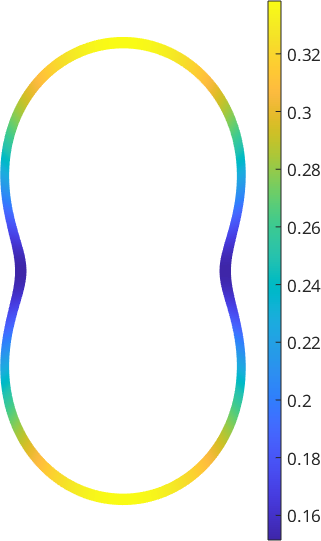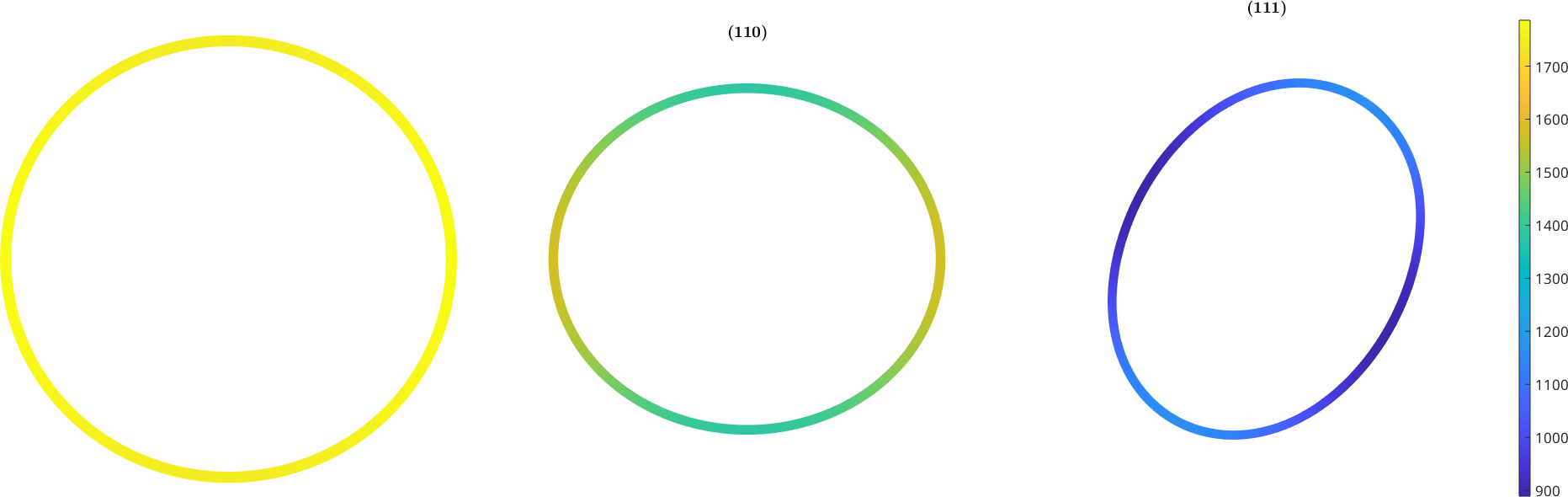Anisotropic Elasticity edit page

The linear theory of ellasticity in anisotropic materials is essentialy based on the fourth order stiffness tensor C. Such a tensor is represented in MTEX by a variable of type stiffnessTensor. Such a variable can either by set up using a symmetric 6x6 matrix or by importing it from an external file. The following examples does so for the stiffness tensor for Olivine

## Hooke's Law

The stiffness tensor tensor of a material is defined as the stress the material expreances for a given strain

Now Hooke's law states that the resulting stress can be computed by

The other way the compliance tensor S = inv(C) translates stress into strain

The ellastic energy of the strain eps can be computed equivalently by the following equations

## Young's Modulus

Young's modulus is also known as the tensile modulus and measures the stiffness of elastic materials. It is computed for a specific direction d by the command YoungsModulus.

If the direction d is ommited Youngs modulus is returned as a spherical function.## Linear Compressibility

The linear compressibility is the deformation of an arbitrarily shaped specimen caused by an increase in hydrostatic pressure and can be described by a second rank tensor. Similar to the Young's modulus it can be computed by the command linearCompressibility for specific directions d or as a spherical function## Poisson Ratio

The rate of compression / decompression in a direction n normal to the pulling direction p is called Poisson ratio.

If we ommit in the call to PoissonRatio the last argument

we again obtain a spherical function. However, this time it is only meaningful to evaluate this function at directions perpendicular to the pulling direction p. Hence, a good way to visualize this function is to plot it as a section in the x/y plane## Shear Modulus

The shear modulus is TODO## Wave Velocities

Since elastic compression and decompression is mechanics of waves traveling through a medium anisotropic compressibility causes also anisotropic waves speeds. The analysis of this anisotropy is explained in the section wave velocities.